# MnDOT Resilient Modulus (Mr)

Testing Protocol and Data Quality Control Criteria

## Testing Protocol and Data Quality Control Criteria

The current MnDOT resilient modulus test, in general, follow the protocol developed from NCHRP 1-28a project. One of the objectives of NCHRP 1-28a was to recommend an enhanced protocol to ASTM, SHRP, AASHTO and NCHRP 1-28 procedures. This protocol was intended to support Mechanistic - Empirical Pavement Design Guide (MEPDG). The protocol can be found in the Appendix 2 of the NCHRP Research Results Digest 285.

MnDOT has made an improvement in the test configuration. The NCHRP 1-28a protocol only uses two LVDTs, but MnDOT requires three on-specimen LVDTs to measure the specimen deformation (Figure 1). The three LVDTs are mounted between two aluminum rings and equally spaced around the specimen to directly measure axial displacement of the specimen. Figure 2 shows the experiment setup. In terms of data acquisition, MnDOT recommends the sampling rate of at least 400 points/second for data acquisition. The baseline values for Mr calculation is determined by taking the average load/deformation values of baseline from the last 60% of a time history cycle.

Mr test of a specimen should represent element responds in field. However, the specimen is not homogenous, the specimen deformation is not always uniform through the specimen height. Also, the testing system could contribute errors to the results, such as system noise. Therefore, controlling Mr data quality is important. NCHRP 1-28 recommends an acceptable two vertical LVDT measurement ratio of 1.1 as a quality control criterion. But we found that this criterion cannot adequately address test quality, such as rotation of the specimen. Based on our testing data, MnDOT has developed a set of data quality control criteria to replace the NCHRP recommended 1.1 ratio criterion. There criteria are Angle of Rotation (AR); Signal-to-Noise ratio (SNR) and Coefficient of Variation of resilient modulus (COV).

##### Angle Of Rotation

AR is used as a measure to control specimen deformation uniformity.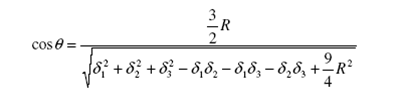δi - recoverable displacement of LVDT "i" (i= 1,2 ,3)

Limit: AR< 0.04o

A detailed documentation of AR criterion can be found in this paper

##### Signal-to-Noise Ratio (SNR)

Noise from measurement devices, such as LVDT, affects data quality, especially when the measured displacement is small. SNR is used to control noise effects on Mr results. SNR is calculated from time history data of each load and displacement measurement.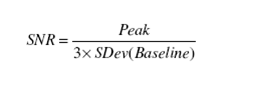Peak – Peak values of load or LVDT from time history data.

Sdev(Baseline) -- standard deviation of baseline valuesμ - mean of baseline values

Y(n) = value at point n

N = total data points

Limit: SNR >= 3, for each LVDT at each cycle of the last 5 cycles used to calculate Mr.

SNR >=10, for load at each cycle of the last 5 cycles used to calculate Mr.

The following are the examples of SNR: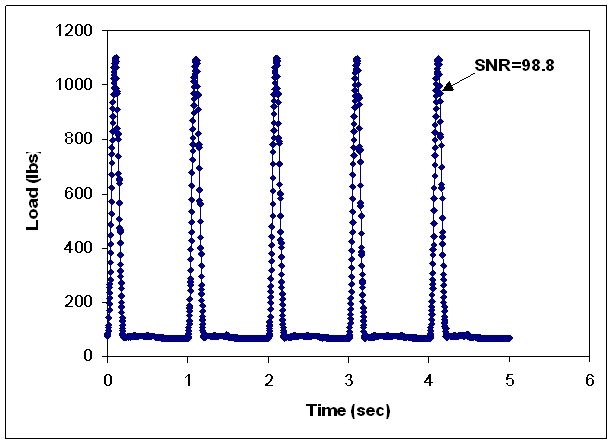##### Coefficient of Variation (COV)

Mr is calculated using each cycle data of the last five cycles in each sequence, Mr values for the last five cycles should be similar. Therefore, it is important to control the variation for each sequence. COV of resilient modulus values is defined as:Sdev – Standard deviation of Mr values during the last five cycles in each sequence.

Average – Average Mr value of the last five cycles.

Limit: COV < 10%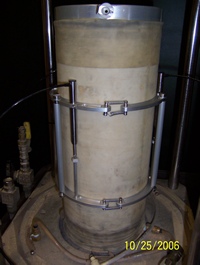Figure 1. LVDT Configuration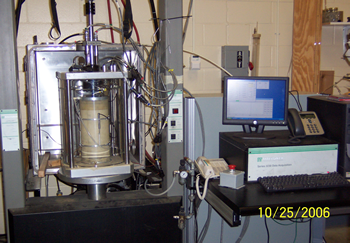Figure 2. Experiment Setup

##### Material Index Tests

The following index tests are generally conducted associated with resilient modulus test: